« Design spectrum of horizontal seismic actions Seismic stresses »

Dynamic analysis και natural periods of structure

The differential equation of motion

The motion of a building model under the seismic ground excitation may be expressed in terms of the vector differential equation: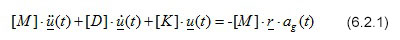where:

 [M], [D], [K] NxN the symmetric and positive definite matrices of mass, damping and stiffness respectively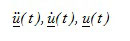Nx1 the vectors of accelerations, velocities and displacements of degrees of freedom of the model r Nx1 the vector of ground motion effect, with unit values at positions of degrees of freedom corresponding to a seismic direction and zero values at rest ag(t) the time function of ground seismic acceleration N the number of degrees of freedom of the model

Eigenvalue analysis

The following transformation is applied to the generalized or natural coordinates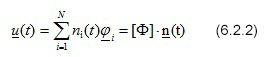where:

n (t) Nx1 vector of generalized functions ni(t)

[ Φ ] NxN normalized matrix of all mode shapes φi

Considering orthogonality of the modes and assuming Rayleigh damping (Bellos, J., 1988, 1989a, 1989b), the problem of solving the system of coupled differential equations 1 ends up to solving N independent differential equations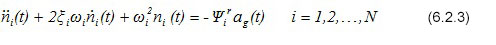Setting: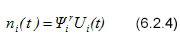equation (6.2.2) is transformed into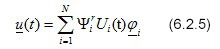and the independent differential equations (6.2.3) are transformed into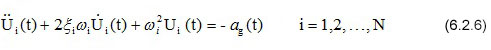Where the natural frequency of each mode is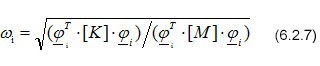the critical damping ratio is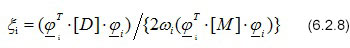And the modal participation factor for vibrations in a specific seismic direction may be expressed as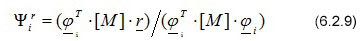Another important quantity is the effective modal mass in a specific seismic direction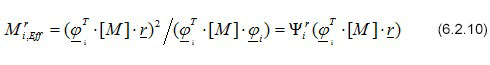The participation of effective modal mass per mode and seismic direction, can be expressed as a percentage of the total mass of the structure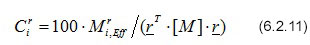In order for an eigenvalue analysis to be sufficient, the sum of percentages of effective modal mass participation for all modes considered, should be significant enough for all primary seismic directions (usually greater than 90%), i.e.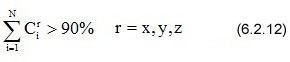Two eigenvalue analysis methods are available in the related software: the swift Lanczos method and the classical Subspace Iteration method.

Modal superposition

Due to the maximum stress and strain resultants being sufficient for the dynamic analysis of buildings rather than the exact time responses themselves, the superposition is based on the ordinates of the seismic accelerations spectrum Rd(T) provided by Seismic Regulations corresponding to discrete values of the natural periods Ti, where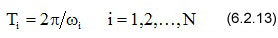Provided that the spectral ordinates of displacements are obtained from the corresponding ordinates of accelerations in a proportion 1/ω i2, the terms of equation (6.2.5) yield that the maximum modal displacements per seismic action are given by the expression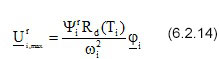The maximum modal values, of course, are usually asynchronous, thus they may not be directly superimposed to provide the total values of the requested stress and strain resultants. To this end, appropriate combination methods are used. According to the most well-known relevant methods, the individual maximum values Vi,max of the components of modal resultants are combined to give the maximum values Vmax:

SRSS (Square Root of Sum of Squares)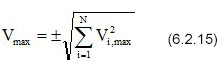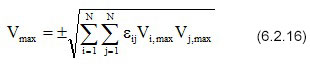where, for damping ratio x as defined by the EC8, the correlation factor of i and j modes is given by the expression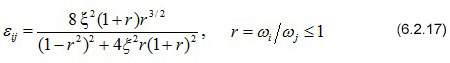Using CQC method is recommended in the related software, as being more general and complete.

« Design spectrum of horizontal seismic actions Seismic stresses »